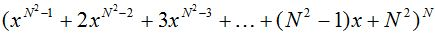Competitions

# Polynomial

Given a polynomialGiven the degree of a polynomial n, calculate the sum of its coefficients modulo 9973 after opening the brackets and reducing such terms.

#### Input

One integer n (1n100) - the degree of the polynom.

#### Output

Print an integer equal to the sum of the coefficients of the polynomial modulo 9973 after opening the brackets and reducing such terms (i.e. the remainder of dividing the sum of the coefficients by 9973).

Time limit 1 second
Memory limit 128 MiB
Input example #1
2

Output example #1
100

Author Andrey Korotkov
Source 2010 XXIII All-Ukrainian Informatics Olympiad, Kiev, March 22 - 26, Round 1